The Quilt Blocks ClipArt gallery offers 100 illustrations of geometric quilt-like patterns that can be used to demonstrate translation and rotation.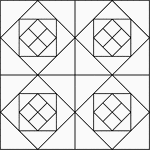### Geometric Block Pattern 1

Geometric pattern for translation and rotation exercises.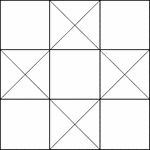### Geometric Block Pattern 10

Geometric pattern for translation and rotation exercises.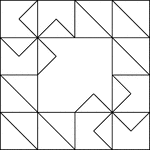### Geometric Block Pattern 100

Geometric pattern for translation and rotation exercises.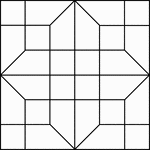### Geometric Block Pattern 101

Geometric pattern for translation and rotation exercises.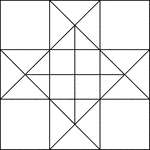### Geometric Block Pattern 11

Geometric pattern for translation and rotation exercises.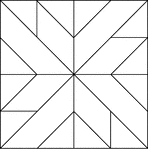### Geometric Block Pattern 12

Geometric pattern for translation and rotation exercises.### Geometric Block Pattern 13

Geometric pattern for translation and rotation exercises.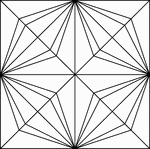### Geometric Block Pattern 14

Geometric pattern for translation and rotation exercises.### Geometric Block Pattern 15

Geometric pattern for translation and rotation exercises.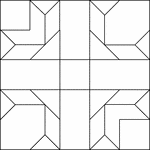### Geometric Block Pattern 16

Geometric pattern for translation and rotation exercises.### Geometric Block Pattern 17

Geometric pattern for translation and rotation exercises.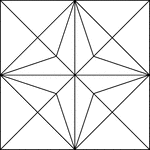### Geometric Block Pattern 18

Geometric pattern for translation and rotation exercises.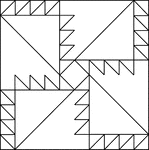### Geometric Block Pattern 19

Geometric pattern for translation and rotation exercises.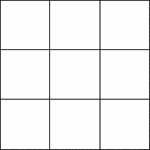### Geometric Block Pattern 2

Geometric pattern for translation and rotation exercises.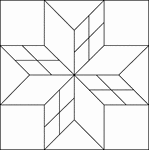### Geometric Block Pattern 20

Geometric pattern for translation and rotation exercises.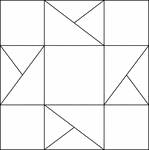### Geometric Block Pattern 21

Geometric pattern for translation and rotation exercises.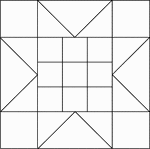### Geometric Block Pattern 22

Geometric pattern for translation and rotation exercises.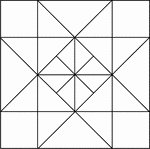### Geometric Block Pattern 23

Geometric pattern for translation and rotation exercises.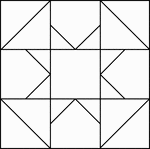### Geometric Block Pattern 24

Geometric pattern for translation and rotation exercises.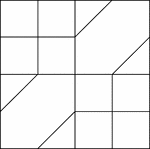### Geometric Block Pattern 25

Geometric pattern for translation and rotation exercises.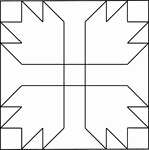### Geometric Block Pattern 26

Geometric pattern for translation and rotation exercises.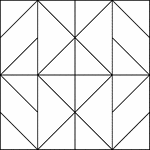### Geometric Block Pattern 27

Geometric pattern for translation and rotation exercises.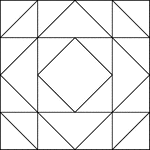### Geometric Block Pattern 28

Geometric pattern for translation and rotation exercises.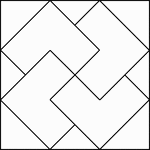### Geometric Block Pattern 29

Geometric pattern for translation and rotation exercises.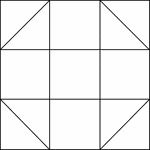### Geometric Block Pattern 3

Geometric pattern for translation and rotation exercises.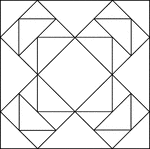### Geometric Block Pattern 30

Geometric pattern for translation and rotation exercises.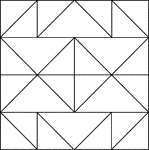### Geometric Block Pattern 31

Geometric pattern for translation and rotation exercises.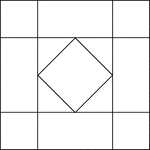### Geometric Block Pattern 32

Geometric pattern for translation and rotation exercises.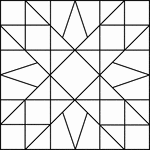### Geometric Block Pattern 33

Geometric pattern for translation and rotation exercises.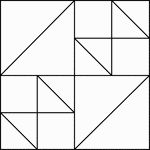### Geometric Block Pattern 34

Geometric pattern for translation and rotation exercises.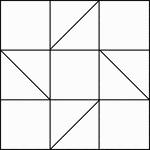### Geometric Block Pattern 35

Geometric pattern for translation and rotation exercises.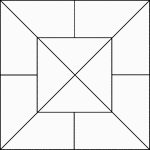### Geometric Block Pattern 36

Geometric pattern for translation and rotation exercises.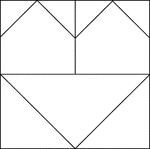### Geometric Block Pattern 37

Geometric pattern for translation and rotation exercises.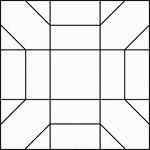### Geometric Block Pattern 38

Geometric pattern for translation and rotation exercises.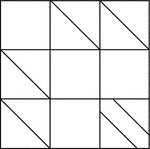### Geometric Block Pattern 39

Geometric pattern for translation and rotation exercises.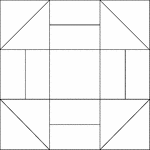### Geometric Block Pattern 4

Geometric pattern for translation and rotation exercises.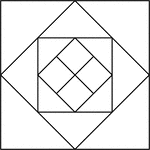### Geometric Block Pattern 40

Geometric pattern for translation and rotation exercises.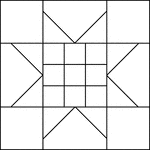### Geometric Block Pattern 41

Geometric pattern for translation and rotation exercises.### Geometric Block Pattern 42

Geometric pattern for translation and rotation exercises.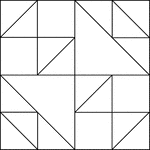### Geometric Block Pattern 43

Geometric pattern for translation and rotation exercises.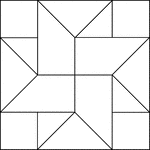### Geometric Block Pattern 44

Geometric pattern for translation and rotation exercises.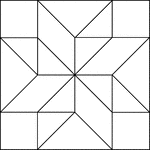### Geometric Block Pattern 45

Geometric pattern for translation and rotation exercises.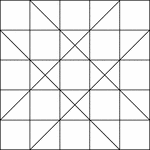### Geometric Block Pattern 46

Geometric pattern for translation and rotation exercises.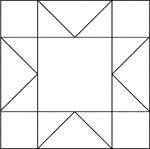### Geometric Block Pattern 47

Geometric pattern for translation and rotation exercises.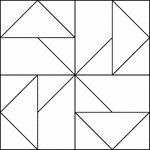### Geometric Block Pattern 48

Geometric pattern for translation and rotation exercises.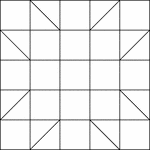### Geometric Block Pattern 49

Geometric pattern for translation and rotation exercises.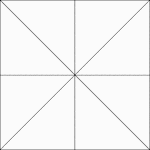### Geometric Block Pattern 5

Geometric pattern for translation and rotation exercises.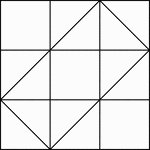### Geometric Block Pattern 50

Geometric pattern for translation and rotation exercises.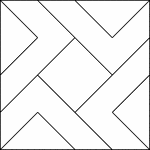### Geometric Block Pattern 51

Geometric pattern for translation and rotation exercises.### Geometric Block Pattern 52

Geometric pattern for translation and rotation exercises.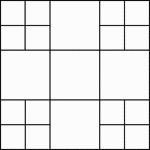### Geometric Block Pattern 53

Geometric pattern for translation and rotation exercises.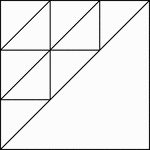### Geometric Block Pattern 54

Geometric pattern for translation and rotation exercises.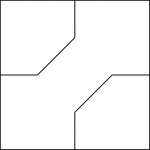### Geometric Block Pattern 55

Geometric pattern for translation and rotation exercises.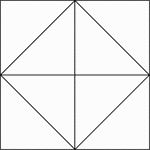### Geometric Block Pattern 56

Geometric pattern for translation and rotation exercises.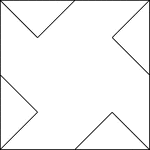### Geometric Block Pattern 57

Geometric pattern for translation and rotation exercises.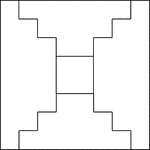### Geometric Block Pattern 58

Geometric pattern for translation and rotation exercises.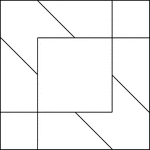### Geometric Block Pattern 59

Geometric pattern for translation and rotation exercises.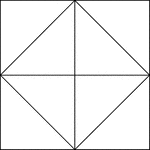### Geometric Block Pattern 6

Geometric pattern for translation and rotation exercises.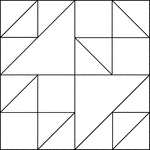### Geometric Block Pattern 60

Geometric pattern for translation and rotation exercises.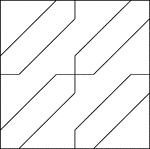### Geometric Block Pattern 61

Geometric pattern for translation and rotation exercises.